# 简述

CSDN学习C语言始于 2022年4月29日

# 第一节 C语言发展史

C标准函数库共有29个头文件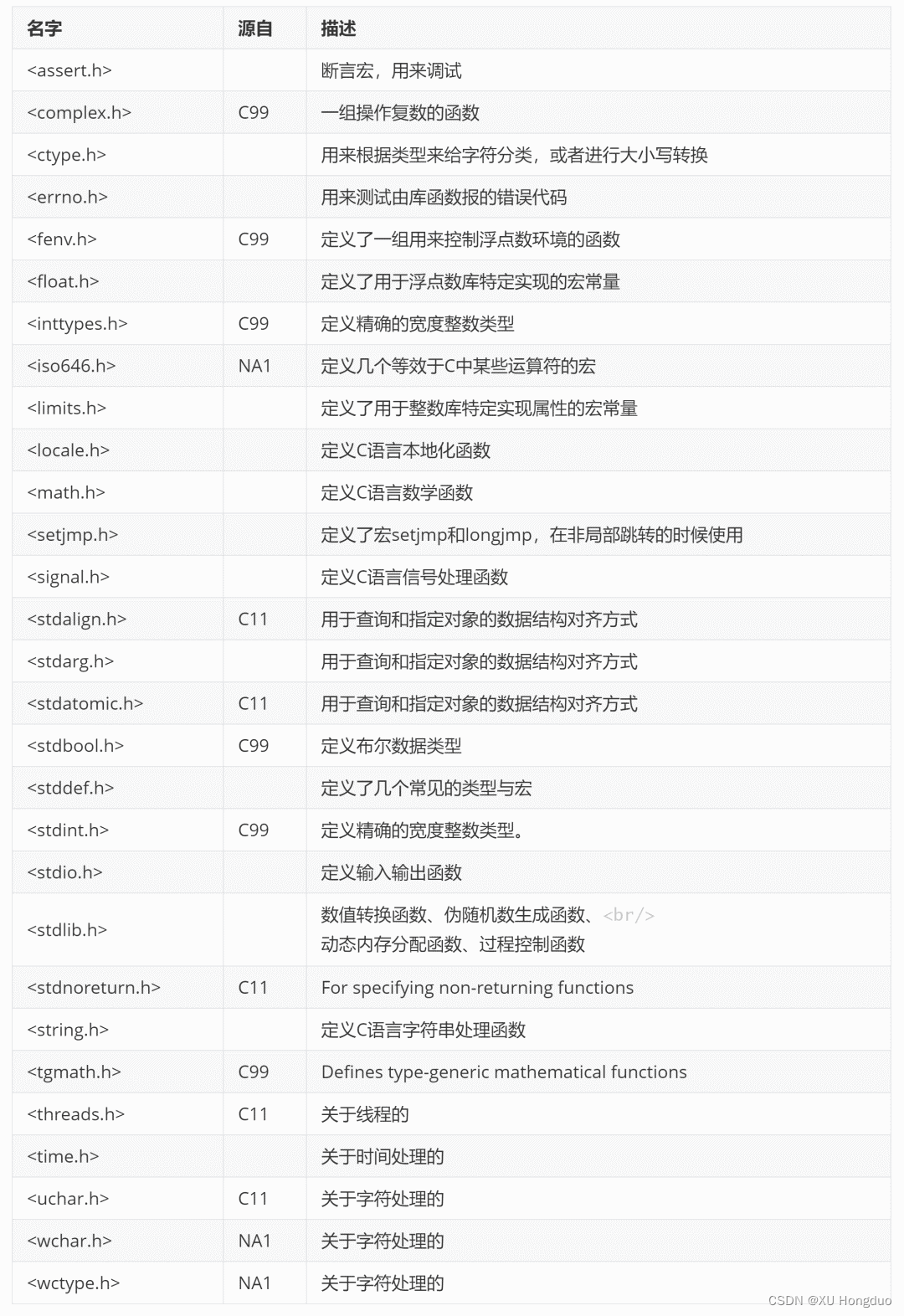# 第二节 数据类型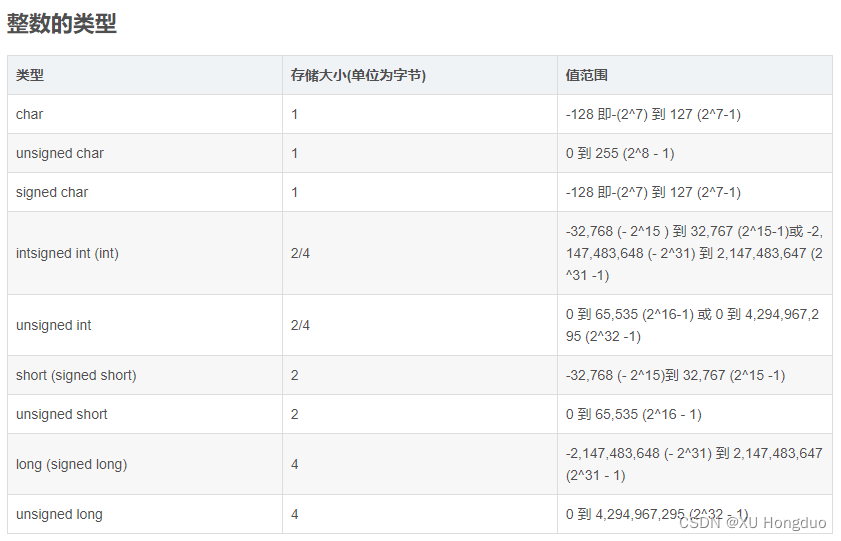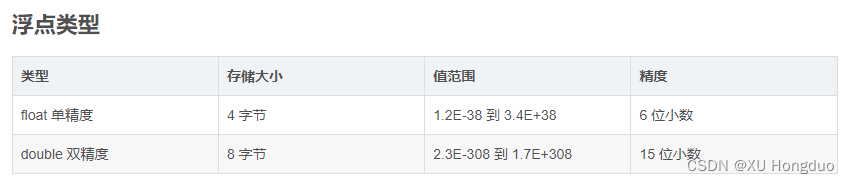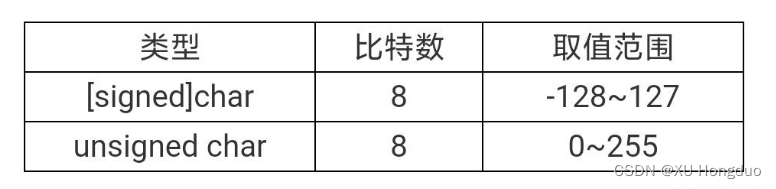# 第三节 C语言中的常量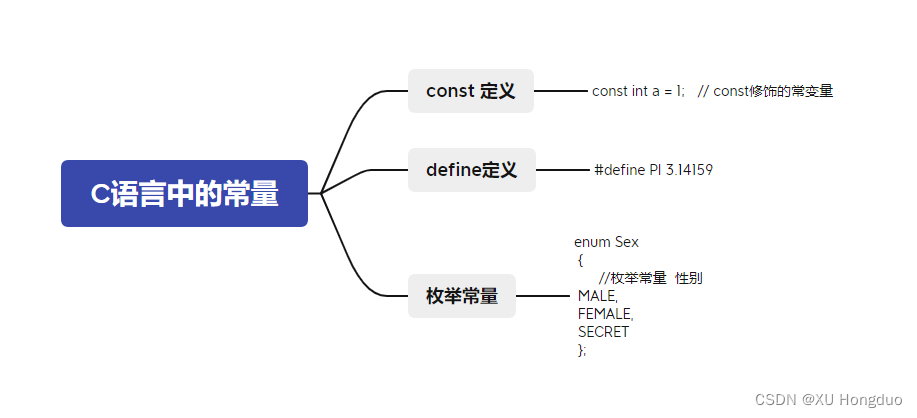// 常量标识策略1
const int a = 1;
// 常量标识策略2
#define PI 3.14159
// 常量标识策略3：枚举
enum Sex
{
//枚举常量     性别
MALE,
FEMALE,
SECRET
};



enum colorname
{red,yellow,blue,white,black};
enum colorname color;


color=red;
color=white;


color=green;
color=orange;


# 第四节 两种赋值运算符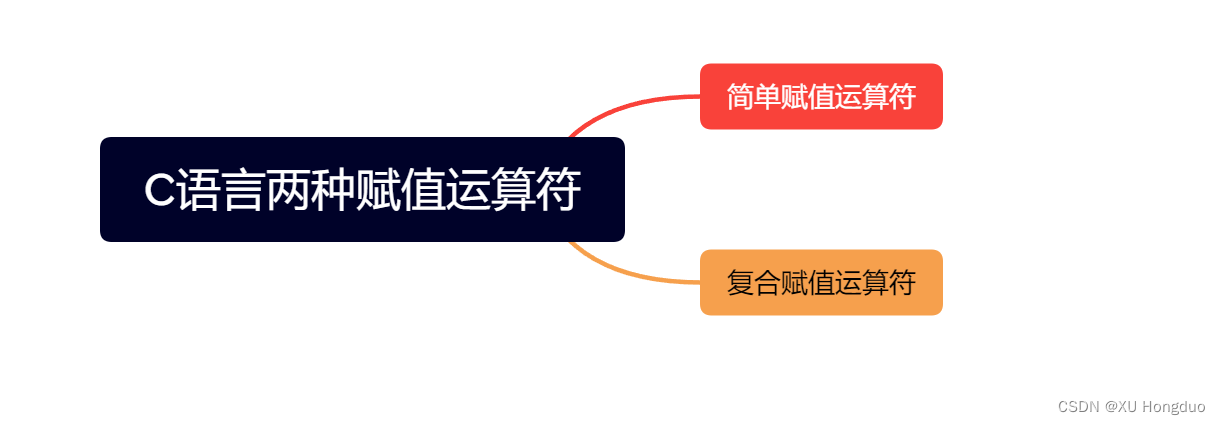#include <stdio.h>

int main()
{
int i = 0;
int j = 1;
i = j;
printf("%d\n",i);
}


#include <stdio.h>

int main()
{
int i = 0;
float j = 72.99;
i = j;
printf("%d\n",i);
}


#include <stdio.h>

int main()
{
int i = 10;
float j = 72.99;
j = i;
printf("%f\n",j);
}


# 第五节 算数运算符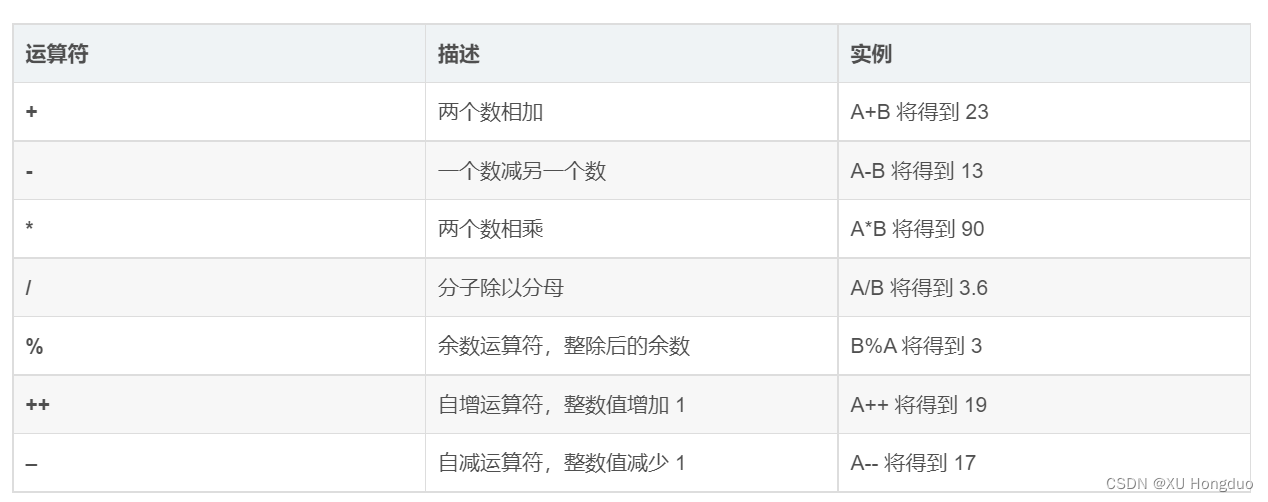i++i–

#include <stdio.h>

int main(int argc, char** argv)
{
int num, count = 0;

printf("请输入一个正整数：");
scanf("%d", &num);

while (num > 0)
{
num /= 10;
int a = ++count;
printf("%d\n",a);
}

printf("位数为：%d", count);
return 0;
}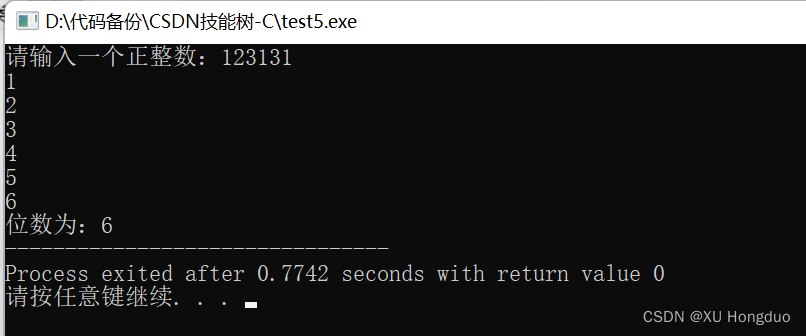++count 换成 count++ :

#include <stdio.h>

int main(int argc, char** argv)
{
int num, count = 0;

printf("请输入一个正整数：");
scanf("%d", &num);

while (num > 0)
{
num /= 10;
int a = count++;	//++count换成了count++
printf("%d\n",a);
}

printf("位数为：%d", count);
return 0;
}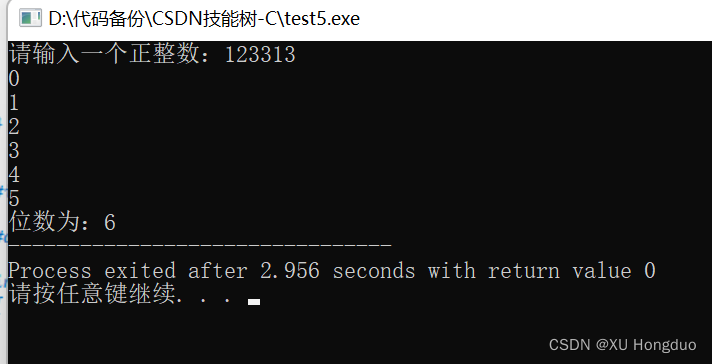# 第六节 关系运算符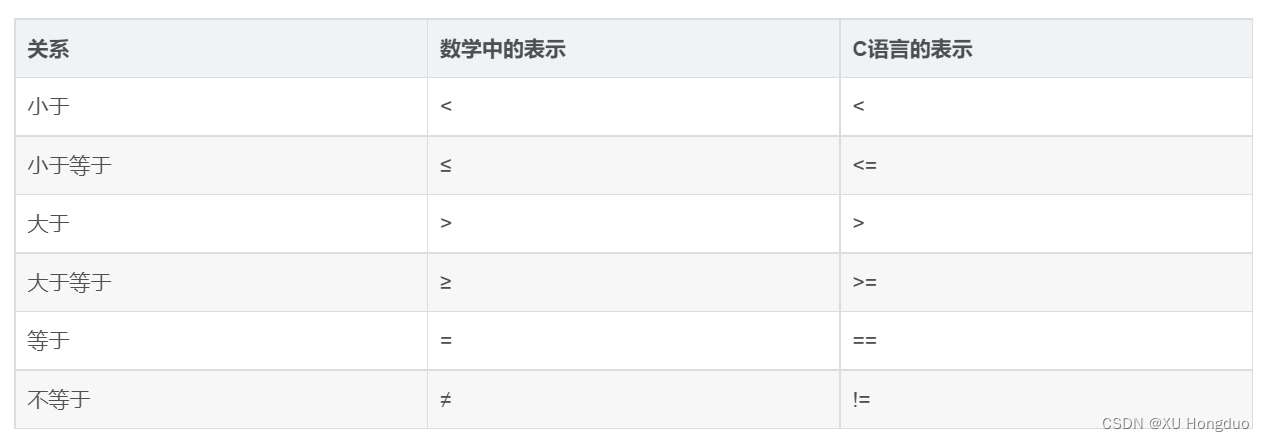# 第七节 特殊的运算符，逗号

#include <stdio.h>
int main()
{
3, 4, 5;//这是一条语句
//把上面这条语句的值赋值给变量a
int a = (3, 4, 5);
printf("a=%d\n", a);
}


a=5

#include <stdio.h>
int main()
{
int a=3, b=4, c=5;
int x=0;
int y=((x=a+b),(b+c));
printf("y=%d\n", y);

}


y=9

# 第八章 三目运算符

## 最简的三目运算符

• 三目运算符属于条件运算符，可与 if 判断函数类比。
• 其运算规则是：先计算表达式1中内容，如果表达式1中内容为真，则计算表达式2中内容并返回；若表达式1中内容为假，则计算表达式3中内容并返回。
• 最简单的三目运算符如：
#include<stdio.h>

int main(){
int a,b,c;
a=7;
b=6;
c=(a>b)?a:b;

return 0;
}


#include<stdio.h>

int main(){
int a,b,c;
a=7;
b=6;
if (a>b) c=a;
else c=b;

return 0;
}


## 提高三目运算符复杂度

#include <stdio.h>

int main()
{
int a, b, c, max;
printf("请输入3个整数：");
scanf("%d,%d,%d", &a, &b, &c);

max = a > b ? (a > c ? a : c) : (b > c ? b : c);
printf("3个整数中的最大的是：%d", max);

return 0;
}


max = a > b ? (a > c ? a : c) : (b > c ? b : c);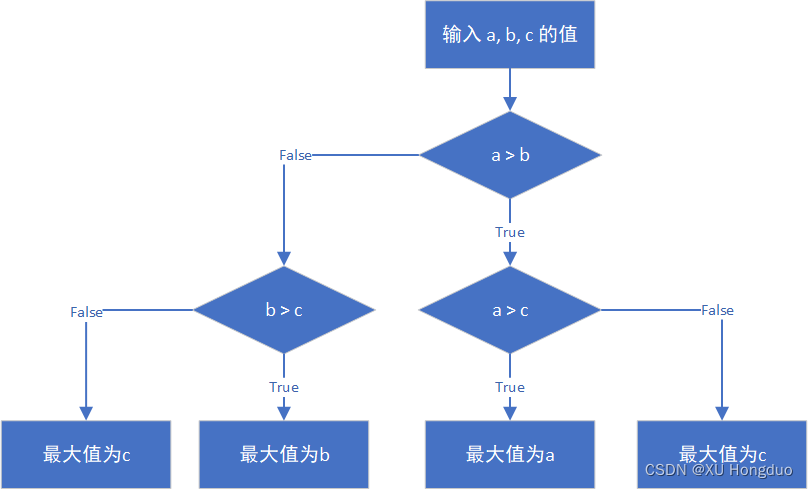## 难理解的三目运算符

#include <stdio.h>

int main()
{
int a, b, c, max;
printf("请输入3个整数：");
scanf("%d,%d,%d", &a, &b, &c);

max = (c > ((a > b) ? a : b)) ? c : ((a > b) ? a : b);
printf("3个整数中的最大的是：%d", max);

return 0;
}


max = (c > ((a > b) ? a : b)) ? c : ((a > b) ? a : b);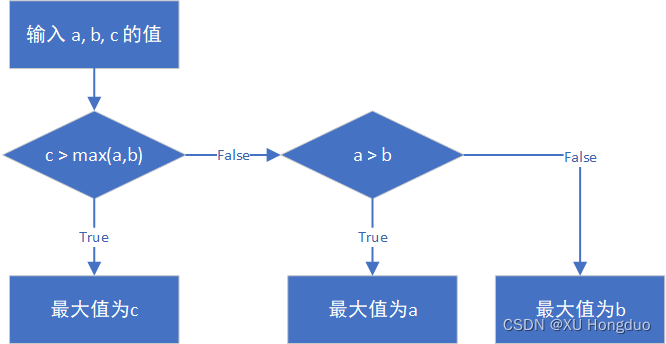# 第十节

04-25208
04-0594
04-071594
07-083434
05-03779
02-071688
06-15758
07-01572
04-21
03-16
10-09
11-02192
11-056599

### “相关推荐”对你有帮助么？

•非常没帮助
•没帮助
•一般
•有帮助
•非常有帮助被折叠的  条评论 为什么被折叠?到【灌水乐园】发言¥2 ¥4 ¥6 ¥10 ¥20余额支付 (余额：-- )扫码支付获取中扫码支付点击重新获取扫码支付1.余额是钱包充值的虚拟货币，按照1:1的比例进行支付金额的抵扣。
2.余额无法直接购买下载，可以购买VIP、C币套餐、付费专栏及课程。余额充值